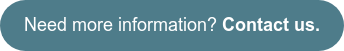# jQuery: Set Scroll Bar to Center on Element-UPDATED

July 3, 2012 | 11:00 AM
If you have a horizontal (or vertical) scroll container and want to set the scroll to center a specific element in the container, you can use the following super simple technique. I'm going to show you how it was derived, because it's important to know why, not simply how.Setup HTML:

`[html]`
`<div class="outer">`
`<div>`
`<ul>`
`<li><div>Alpha</div></li><li><div>Beta</div></li>...<li><div>Omega</div></li>`
`</ul>`
`</div>`
`</div>`
`[/html]`

Setup CSS:

`[css]`
`div.outer {`
`width: 400px; height: 100px; overflow-x: auto; overflow-y: hidden;`
`}`
`div.outer > div {`
`display: table;`
`}`
`ul {`
`display: table-row;`
`}`
`li {`
`display: table-cell; border: 2px solid gray;`
`}`
`li div {`
`width: 120px; height: 120px;`
`}`
`[/css]`

With this setup, we should have a nice horizontally scrolling list of items of all the same width.

``````  |--DIV.outer---------------------------------|         { Hidden Elements }
||--DIV-and UL------------------------------|/....................................|
|||---LI---||---LI---||---LI---||---LI---||-//LI...||...LI...||...LI...||...LI...||
|||        ||        ||        ||        || //     ||        ||        ||        ||
|||        ||        ||        ||        || //     ||        ||        ||        ||
|||--------||--------||--------||--------||-//.....||........||........||........||
||------------------------------------------|/....................................|
|--------------------------------------------|``````

jQuery provides a function, scrollLeft (and scrollTop) that lets you read or set the scroll bar position of an element at the pixel level. So, if we call \$('.outer').scrollLeft() and it returns zero (0) we know the scroll bar is all the way to the left. If the scroll bar is all the way to the right the value of .scrollLeft() will be the width of the inner element minus the width of the container. We will see how this fits in next.

Consider following code:

`[js]`
`var outer = \$('.outer');`
`var inner = \$('.outer > div');`
`outer.scrollLeft( (inner.width() - outer.width()) / 2);`
`[/js]`

This code will set the scroll bar to the center of the inner content. But lets examine why. We know the minimum value for scrollLeft is zero, and the maximum is inner.width() - outer.width(). So, half way is easily half the maximum. Simple! Now, this can still fail. If your inner element has padding or a margin or border then the value of .width() will be incorrect. You should use .outerWidth(true), which includes the border, padding and (because we passed true) the margin.

So, how will we get it to center on an arbitrary element in the list? We use a very similar method to the one above, except we also need the width of the target element.

Here is the code to center a scrolling container on a target element inside:

`[js]`
`var target = \$('li.active'); //Lets assume one element has the class active, it is the one we want to center`
`var containerWidth = outer.width();`
`var targetWidth = target.outerWidth(true);`
`var targetIndex = target.index(); //Tells us what position this is in. Useful in calculations`

`var leftScreenOffset = (containerWidth - targetWidth) / 2; //See Note 1 below`
`var leftSiblingOffset = targetWidth * targetIndex; //See Note 2 below`

`var scrollValue = leftSiblingOffset - leftScreenOffset;`
`outer.scrollLeft(Math.max(0, scrollValue)); our target is now centered.`
`[/js]`

• Note 1: This should be familiar. This is how many pixels the target needs to be to the right
to appear centered. From our CSS we know that containerWidth should be 300 and targetWidth 120.
So, leftScreenOffset = 400-120 /2 = 140
• Note 2: If targetIndex=3, there are 3 other elements preceding our target (the first element is index zero (0) as with most indexing). From our CSS targetWidth should be 120. This calculation lets
us know exactly how many pixels preceded our target. 3 * 120 = 360 pixels.

So, why does leftSiblingOffset - leftScreenOffset center our target? We know that:

leftSiblingOffset = 360 -> The total number of pixels between the target's left edge and the left edge of it's parent.

leftScreenOffset = 140 -> The total number of pixels we need to show to the left of the target to center it.

That means we need to hide leftSiblingOffset - leftScreenOffset, or 360 - 140, or 220 pixels off the screen.

UPDATE: The example below has been updated to reflect the proper technique

• Alpha
• Beta
• Gamma
• Delta
• Epsilon
• Zeta
• Eta
• Theta
• Iota
• Kappa
• Lamda
• Mu
• Nu
• Xi
• Omikron
• Pi
• Rho
• Sigma
• Tau
• Upsilon
• Phi
• Chi
• Psi
• Omega

Super simple stuff! This technique only works if the width of each child element is homogeneous. If they are variable it is still relatively simple to center. You just need to add up the widths of all siblings preceding your target element. Here are two different versions of the code, the second working with variable widths.

```[js]
function centerItFixedWidth(target, outer){
var out = \$(outer);
var tar = \$(target);
var x = out.width();
var y = tar.outerWidth(true);
var z = tar.index();
out.scrollLeft(Math.max(0, (y * z) - (x - y)/2));
}```
```function centerItVariableWidth(target, outer){
var out = \$(outer);
var tar = \$(target);
var x = out.width();
var y = tar.outerWidth(true);
var z = tar.index();
var q = 0;
var m = out.find('li');
//Just need to add up the width of all the elements before our target.
for(var i = 0; i < z; i++){
q+= \$(m[i]).outerWidth(true);
}
out.scrollLeft(Math.max(0, q - (x - y)/2));
}
[/js]hbspt.cta._relativeUrls=true;hbspt.cta.load(347829, '4eff913d-bfae-48af-bea1-29a0a9c6de36', {});

```

## A Great Offer, Just a Click Away

Lorem ipsum dolor sit amet, consectetur adipiscing elit